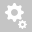##Recently viewed tickets# [ANALYSIS]     Cable element is automatically transformed to equivalent truss element for linear analysis. What does this message imply?

A cable element is automatically transformed into an equivalent truss element in the cases of linear analysis and is considered as an elastic
catenary cable element only in case of geometric nonlinear analysis.

Cable is a three-dimensional line element, which is capable of transmitting only axial tension force. Cables are inherently non-linear and the cable
element stiffness varies with internal tension forces.This change in stiffness could not be captured with linear analysis and hence a geometric nonlinear analysis is often required. To over come this, midas Civil adopts the concept of Equivalent Truss Elements for linear analysis, which
incorporates stiffness change due to sag effects of cable as well.

The stiffness of an equivalent truss element is composed of the usual elastic stiffness and
the stiffness resulting from the sag, which depends on the magnitude of the tension force.
The following expressions calculate the stiffness:E : modulus of elasticity
A : cross-sectional area
L : length
w : weight per unit length
T : tension force

In cable bridges, when we try to estimate cable pretension using the ‘Unknown Load Factor’ function,
we formulate a linear model with cables modelled as equivalent truss elements.
Creation date: 6/22/2016 6:53 PM ()      Updated: 9/5/2017 9:45 AM ()